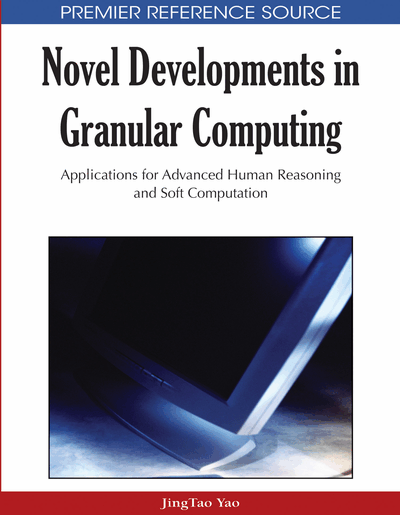# Rule Extraction and Rule Evaluation Based on Granular Computing

Jiye Liang (Shanxi University, P.R. China), Yuhua Qian (Shanxi University, P.R. China) and Deyu Li (Shanxi University, P.R. China)
DOI: 10.4018/978-1-60566-324-1.ch009

## Abstract

In rough set theory, rule extraction and rule evaluation are two important issues. In this chapter, the concepts of positive approximation and converse approximation are first introduced, which can be seen as dynamic approximations of target concepts based on a granulation order. Then, two algorithms for rule extraction called MABPA and REBCA are designed and applied to hierarchically generate decision rules from a decision table. Furthermore, to evaluate the whole performance of a decision rule set, three kinds of measures are proposed for evaluating the certainty, consistency and support of a decision-rule set extracted from a decision table, respectively. The experimental analyses on several decision tables show that these three new measures are adequate for evaluating the decision performance of a decision-rule set extracted from a decision table in rough set theory. The measures may be helpful for determining which rule extraction technique should be chosen in a practical decision problem.
Chapter Preview
Top

## 1. Introduction

Granular computing (GrC) is a new active area of current research in artificial intelligence, and is a new concept and computing formula for information processing. It has been widely applied to many branches of artificial intelligence such as problem solving, knowledge discovery, image processing, semantic web services [18, 29-31, 37].

As follows, for our further development, we briefly review research on GrC. In 1979, the problem of fuzzy information granules was introduced by Zadeh. Then, in [47, 48] he introduced a concept of granular computing, as a term with many meanings, covering all the research of theory, methods, techniques and tools related to granulation. He identified three basic concepts that underlie the process of human cognition, namely, granulation, organization, and causation. “Granulation involves decomposition of whole into parts, organization involves integration of parts into whole, and causation involves association of causes and effects”. Some authors [37, 45] examined granular computing in connection with the theory of rough sets. Yao [45, 46] suggested the use of hierarchical granulations for the study of stratified rough set approximations and studied granular computing through using neighborhood systems. The theory of quotient space had been extended into the theory of fuzzy quotient space based on fuzzy equivalence relation . Liang and Shi [14, 15] established the relationship among knowledge granulation, information entropy, granularity measure and rough entropy in information systems. Liang and Qian  developed an axiomatic approach to knowledge granulation in information systems. Granular computing mainly has three important models: (1) computing with words, (2) rough set theory, and (3) quotient space theory. As applications of granular computing, in this chapter, we focus on rule extraction and rule evaluation based on granular computing.

In the view of granular computing, a target concept described by a set is always characterized via the so-called upper and lower approximations under static granulation in rough set theory, and a static boundary region of the concept is induced by the upper and lower approximations [22-25]. However, through this mechanism, we cannot improve the approximation measure of a rough set and more clearly analyze the approximation structure of a rough set [34, 38]. Based on these conclusions, one objective of this chapter is to establish two kinds of structures of the approximation of a target concept by introducing a notion of a granulation order, called positive approximation and converse approximation, and to apply them to rule extracting from decision tables.

## Complete Chapter List

Search this Book:
Reset